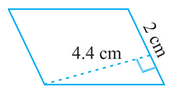# Q. 1.     Find the area of the following parallelograms:R Riya

We know that

Area of parallelogram

Here,

Base of parallelogram = 2 cm

and

Height of parallelogram = 4.4 cm

Therefore, area of parallelogram is

Exams
Articles
Questions